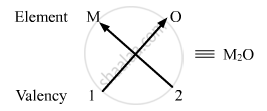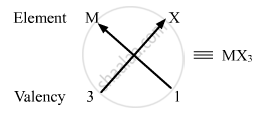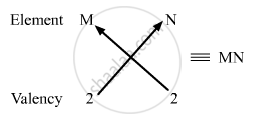# Based on the Group Valency of Elements State the Formula Lot the Following Giving Justification for Each - Science

Based on the group valency of elements state the formula lot the following giving justification for each :-

(i) Oxides of 1st group elements,

(ii) Halides of the elements of group 13, and

(iii) Compounds formed when an element of group 2 combines with, an element of group 16.

#### Solution

(i) Valency of group 1 elements: 1

Valency of oxygen: 2

Oxides of group 1 elements:Formula of the oxides of group 1 is M2O, where M is the group 1 element and O is oxygen.

(ii) Valency of group 13 elements: 3

Valency of halogens: 1

Halides of group 13 elements:Formula of the halides of group 13 is MX3, where M is the group 13 element and X is halogen.

(iii) Valency of group 2 elements: 2

Valency of group 16 elements: 2

Compounds of group 2 and group 16 elements:Formula of the compounds of group 2 and 16 is MN, where M is the group 2 element and N is the group 16 element.

Concept: Periodic Properties
Is there an error in this question or solution?